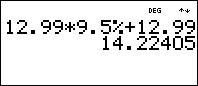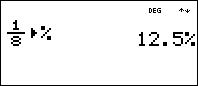# Knowledge Base

## Solution 20309: Calculating Percents and Percent Conversions Using TI-30XS/B MultiView™ and TI-34 MultiView™ Scientific Calculators.

### How do I calculate percents and percent conversions using the TI-30XS/B MultiView and TI-34 MultiView calculators?

The examples below will demonstrate how to calculate percents and perform percent conversions using the TI-30XS/B MultiView and TI-34 MultiView calculators.

Example: If a music CD cost $12.99 and the sales tax is 9.5%, what is the total cost for the CD? If using the TI-30XS/B MultiView: 1) Input 12.99. 2) Press the [x] or multiplication key. 3) Input 9.5 and press [2nd] [%]. 4) Press the [+] or addition key. 5) Input 12.99 and press [enter]. If using the TI-34 MultiView: 1) Input 12.99. 2) Press the [x] or multiplication key. 3) Input 9.5 and press the [%] key. 4) Press the [+] or addition key. 5) Input 12.99 and press [enter].The total cost for the music CD is$14.22.

Example: Convert 1/8 into a percentage.

1) Input 1, press the [n/d] key, then input 8.
2) Press the [] key to move the cursor to the outside of the fraction.
3) Press [2nd] [%] [enter].1/8 as a percentage is 12.5%.

Please see the TI-30XS/B MultiView and TI-34 MultiView guidebooks for additional information.